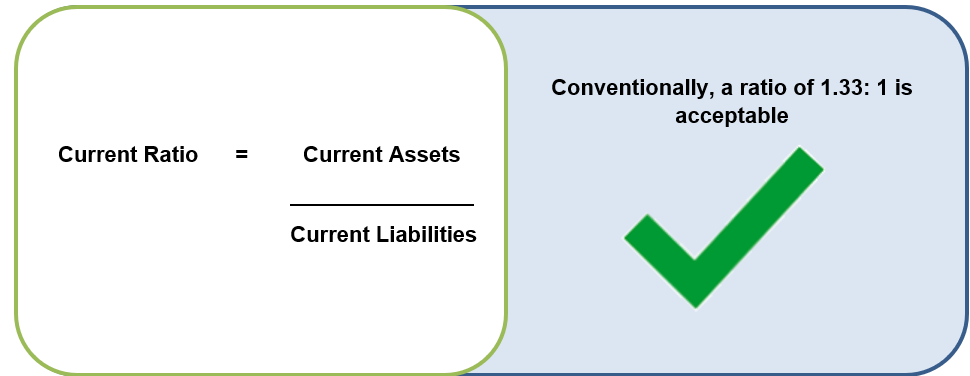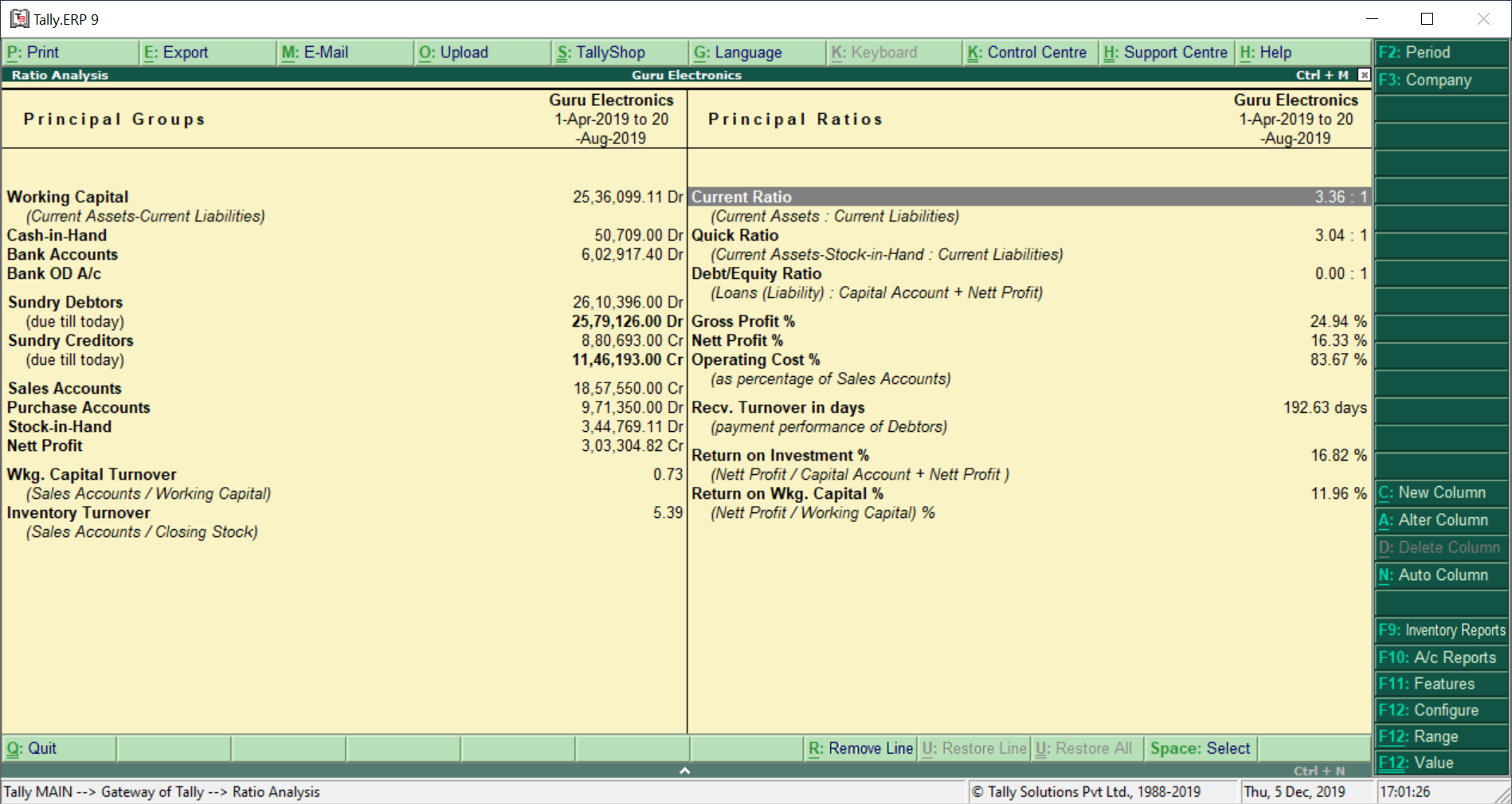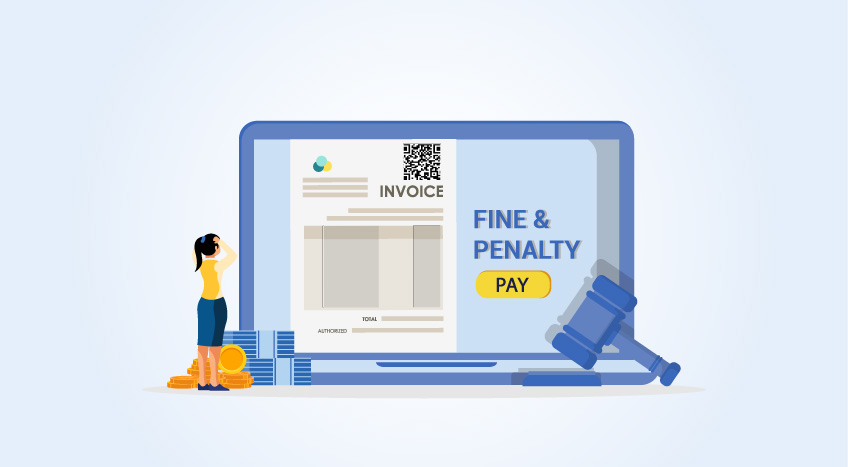# Current Ratio – Formula & How Current Ratio Works with Example

##### Yarab A|Updated on: May 18, 2022

Ratio analysis is a study that determines and interprets numerical relationship based on financial statements. It is with the help of these techniques; the accounting ratios are derived from the financial statements.

Before we understand the current ratio, we need to know about liquidity ratios. Liquidity ratio analyses the short-term financial position of the firm to meet its short-term commitments (Current Liabilities) out of its short-term resources (Current Assets). It is also called as ‘solvency ratios.

In order to figure out the liquidity of the firm, the business uses the following techniques:

• Current ratio
• Liquidity ratio or quick asset ratio or acid test ratio

Let’s understand the current ratio and its significance in a business

## What is current ratio?

Current ratio refers to a technique that measures the capability of a business to meet its short-term obligations that are due within a year. The current ratio considers the weight of the total current assets versus the total current liabilities.

In simple words, it illuminates how a business can maximize the liquidity of its current assets to settle debt and payables.

## How to calculate current ratio?

Going through the definition of the current ratio which is all about how much current assets are available to meet the company’s short-term debt (current liabilities), you arrive the following formula to calculate the current ratio.In the above formula, the current ratio is derived by dividing current assets by current liabilities.

If you are curious to know the components of short-term assets and short-term liabilities, you should read our articles on Current Assets and Current Liabilities.

Are you wondering how to read 1.33:1? We have covered in the next section.

## Significance of current ratio in a business

The current ratio indicates the availability of current assets in rupee for every one rupee of current liability. A ratio greater than 1 implies that the firm has more current assets than a current liability.

For example, a current ratio of 1.33:1 indicates 1.33 assets are available to meet the short-term liability of Rs. 1.

 Current ratio indicators 2:1 1.33:1 <1:1 Ideal and considered to be  satisfactory Considered as an acceptable current ratio Considered as Poor ratio and if it prolongs for a longer time, it is a warning

The significance of the current ratio is extremely important when a business is looking for financial help from the banks and financial institutions. This is one of key indicator which banks and financial institutions look for before lending the credit.

## Consequences of higher and lower current ratio

While considering the ideal current ratio of 2:1, anything higher or lower indicates the following

• Higher current ratio indicates under trading and overcapitalization.
• A lower current ratio indicates over trading and undercapitalization.

## Current ratio example with calculations

Let us calculate current ratio of Kiran Enterprises considering the below example

 Particulars Amount (Rs.) Particulars Amount (Rs) Bank 14,500 Land and Building 2,02,000 Expenses Paid in advance 17,500 Stock 70,250 Creditors 1,03,500 Debtors 1,32,750 Bills receivables 7,250 Plant and Machinery 1,38,000 12% Debentures 64,500 Loan from Director (Payable after 3 years) 1,00,000 Equity Share Capital 2,52,000 Profit and Loss Account (Cr) 57,250

The formula to arrive at the current ratio is as below

Current Ratio       =    Current Assets

______________

Current Liabilities

So, to apply the formula, you need to know the total of current assets and current liabilities. Let’s figure this out first using the information from the above table.

 Current Assets Current Liabilities Bank 14,500 Sundry Creditors 1,03,500 Prepaid Expenses 17,500 Bills Receivables 7,250 Sundry debtors 1,32,750 Total 1,72,000 Total 1,03,500

Now apply the formula to calculate current ratio.

Current Ratio        =      1,72,000

_________

1,03,500

=    1.662

A current ratio of Kiran Enterprises is 1.662: 1 which implies the firm has Rs. 1.662 of assets to cover every Rs. 1 in liability.

## How do businesses prepare ratio analysis?

Ratio analysis helps the business owners to get the pulse of the organization quickly through ratios. Ratio Analysis acts as a dashboard of an organization in which all the key factors of a business is summarized in ratios.

While it is an important tool for business owners or decision makers but the time and efforts to determine such ratios is time-consuming. To mitigate this challenge and leverage the insights from ratio analysis, most of the businesses are using accounting software to generate such reports automatically.Ratio Analysis Generated using Tally.ERP 9

## Why use the current ratio formula?

Current ratio refers to the liquidity ratio that gauges an organization's capability to pay off short-term debts. It enables investors and analysts to understand how the venture is performing and can maximize the current assets on its balance sheet to fulfil its existing debt and payables.

## How the current ratio works?

The formula to calculate the current ratio is by dividing a company's current assets by its current liabilities.

## Limitations of the Current Ratio

One of the immediate limitations of the current ratio is that the ratio is not a satisfactory indicator to gauge a company's liquidity. A company cannot just depend on the current ratio since it provides very few details about its working capital.

## FAQs

### What is a good current ratio?

While the spectrum of a good current ratio differs basis the exact industry type, a ratio between 1.5 and 3 is considered healthy.

### Why high current ratio is bad?

Although a high current ratio is considered good, however, if the current ratio is too high, for instance, above 2, it might be that the organization is unable to utilize its current assets effectively.

### How do you fix a high current ratio?

Here are some of the ways to reduce high current ratio:

• Increase short term loans
• Expend cash optimally
• Leaner working capital model

Language

#### Latest BlogsBoost growth with affordable accounting. Take control of your finances today!